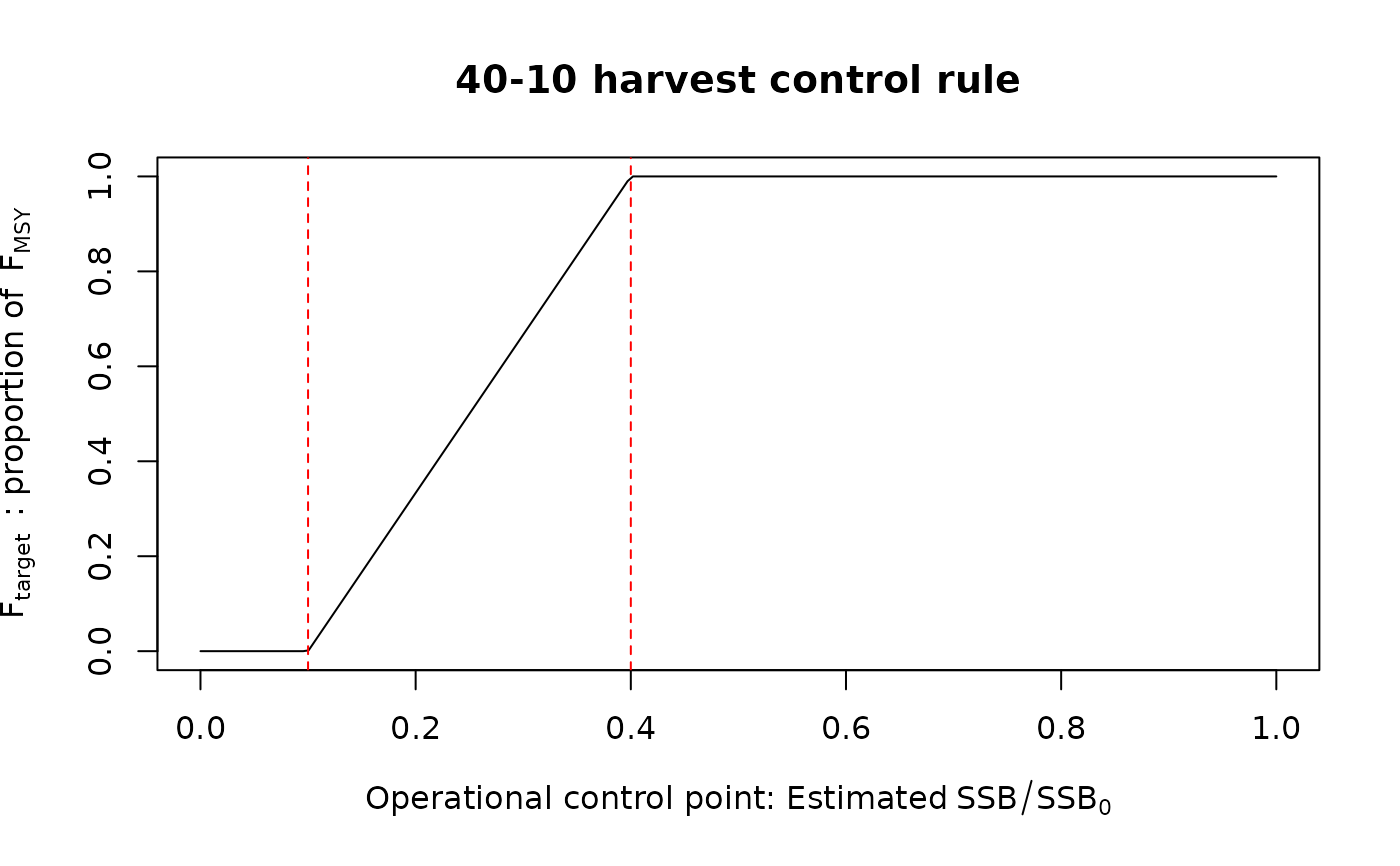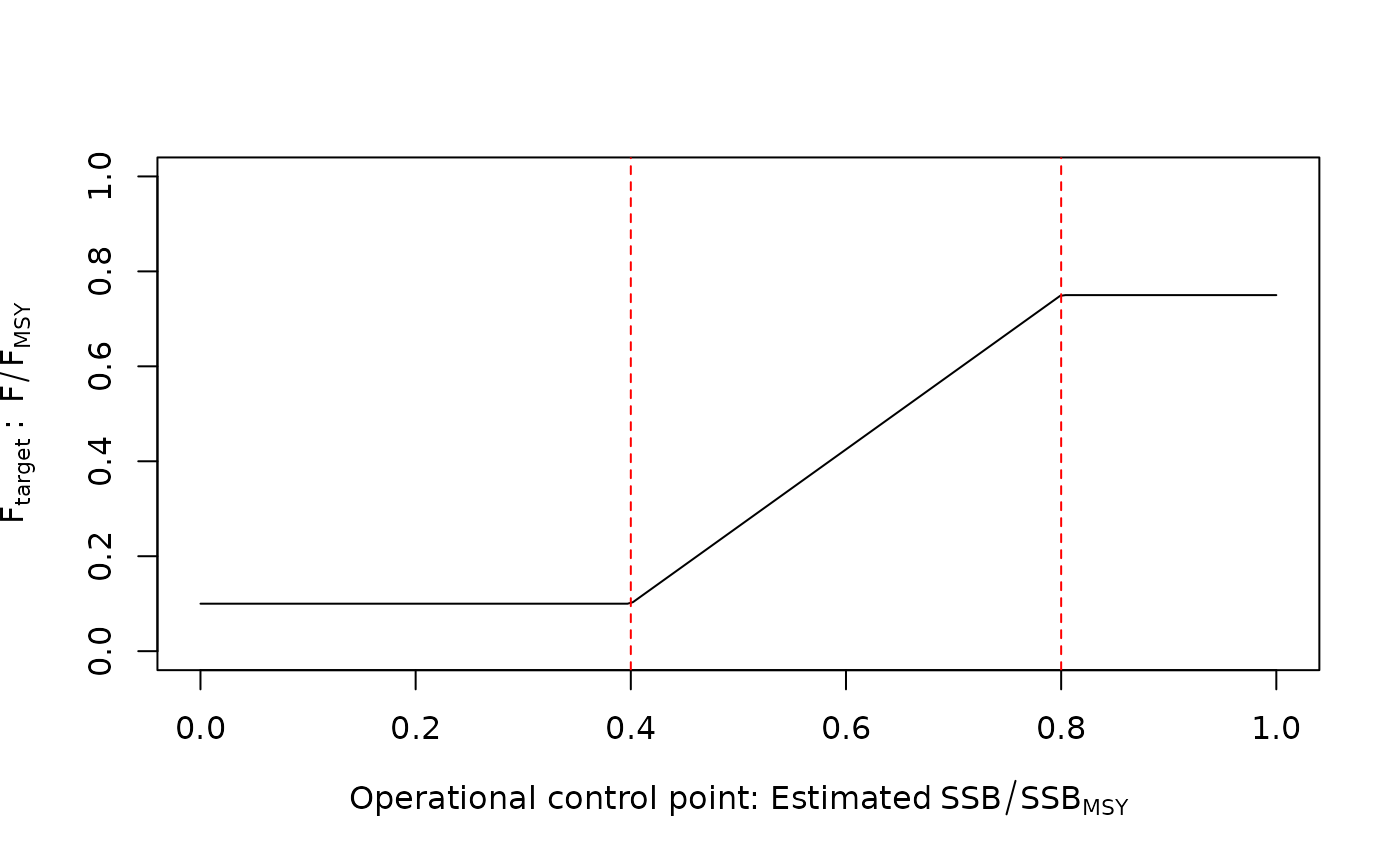An output control rule with a ramp that reduces the target F (used for the TAC recommendation) linearly as a function of an operational control point (OCP) such as spawning depletion or spawning biomass. The reduction in F is linear when the OCP is between the target OCP (TOCP) and the limit OCP (LOCP). The target F is maximized at or above the TOCP. Below the LOCP, the target F is minimized. For example, the TOCP and LOCP for 40% and 10% spawning depletion, respectively, in the 40-10 control rule. Ftarget is FMSY above the TOCP and zero below the LOCP. This type of control rule can generalized with more control points (>2) in HCR_segment. Class HCR objects are typically used with function make_MP.

HCR_ramp(
Assessment,
reps = 1,
OCP_type = c("SSB_SSB0", "SSB_SSBMSY", "SSB_dSSB0", "F_FMSY", "F_F01", "F_FSPR"),
Ftarget_type = c("FMSY", "F01", "Fmax", "FSPR", "abs"),
LOCP = 0.1,
TOCP = 0.4,
relF_min = 0,
relF_max = 1,
SPR_OCP = 0.4,
SPR_targ = 0.4,
...
)

HCR40_10(Assessment, reps = 1, Ftarget_type = "FMSY", SPR_targ = 0.4, ...)

HCR60_20(Assessment, reps = 1, Ftarget_type = "FMSY", SPR_targ = 0.4, ...)

HCR80_40MSY(Assessment, reps = 1, Ftarget_type = "FMSY", SPR_targ = 0.4, ...)

## Arguments

Assessment

An object of class Assessment with estimates of FMSY or UMSY, vulnerable biomass, and spawning biomass depletion in terminal year.

reps

The number of stochastic samples of the TAC recommendation.

OCP_type

The type of operational control points (OCPs) for the harvest control rule used to determine the reduction in F. See below.

Ftarget_type

The type of F used for the target fishing mortality rate. See below.

LOCP

Numeric, the limit value for the OCP in the HCR.

TOCP

Numeric, the target value for the OCP in the HCR.

relF_min

The relative value of Ftarget (i.e., as a proportion) if OCP < LOCP.

relF_max

The relative value of Ftarget if OCP > TOCP.

SPR_OCP

The value of spawning potential ratio for the OCP if OCP_type = "F_FSPR". By default, 0.4 (F40%).

SPR_targ

The target value of spawning potential ratio if Ftarget_type = "FSPR". By default, 0.4 (F40%).

...

Miscellaneous arguments.

## Value

An object of class Rec with the TAC recommendation.

## Details

The catch advice is calculated using the catch equation of the corresponding assessment. See Assessment@forecast$catch_eq, a function that returns the catch advice for a specified Ftarget. Operational control points (OCP_type) The following are the available options for harvest control rule inputs, and the source of those values in the Assessment object: • Default "SSB_SSB0": Spawning depletion. Uses the last value in Assessment@SSB_SSB0 vector. • "SSB_SSBMSY": Spawning biomass relative to MSY. Uses the last value in Assessment@SSB_SSBMSY vector. • "SSB_dSSB0": Dynamic depletion (SSB relative to the historical reconstructed biomass with F = 0). Uses the last value in Assessment@SSB/Assessment@TMB_report$dynamic_SSB0.

• "F_FMSY": Fishing mortality relative to MSY. Uses the last value in Assessment@F_FMSY.

• "F_F01": Fishing mortality relative to F_0.1 (yield per recruit), calculated from the data frame in Asesssment@forecast[["per_recruit"]].

• "F_FSPR": Fishing mortality relative to F_SPR "SPR_OCP", calculated from the data frame in Asesssment@forecast[["per_recruit"]].

Fishing mortality target (Ftarget_type)

The type of F for which the corresponding catch is calculated in the HCR is specified here. The source of those values in the Assessment object is specified:

• Default "FMSY": Fishing mortality relative to MSY. Uses the value in Assessment@FMSY.

• "F01": Fishing mortality relative to F_0.1 (yield per recruit), calculated from the data frame in Asesssment@forecast[["per_recruit"]].

• "Fmax": Fishing mortality relative to F_max (maximizing yield per recruit), calculated from the data frame in Asesssment@forecast[["per_recruit"]].

• "FSPR": Fishing mortality relative to F_SPR "SPR_targ", calculated from data frame in Asesssment@forecast[["per_recruit"]].

• "abs": Fishing mortality is independent of any model output and is explicitly specified in relF.

## Functions

• HCR_ramp(): Generic ramped-HCR function where user specifies OCP and corresponding limit and target points, as well as minimum and maximum relative F target.

• HCR40_10(): Common U.S. west coast control rule (LOCP and TOCP of 0.1 and 0.4 spawning depletion, respectively)

• HCR60_20(): More conservative than HCR40_10, with LOCP and TOCP of 0.2 and 0.6 spawning depletion, respectively).

• HCR80_40MSY(): 0.8 and 0.4 SSBMSY as the LOCP and TOCP, respectively.

## Author

Q. Huynh & T. Carruthers

## Examples

# 40-10 linear ramp
Brel <- seq(0, 1, length.out = 200)
plot(Brel, HCRlin(Brel, 0.1, 0.4),
xlab = expression("Operational control point: Estimated"~SSB/SSB),
ylab = expression(F[target]~~": proportion of"~~F[MSY]),
main = "40-10 harvest control rule", type = "l")
abline(v = c(0.1, 0.4), col = "red", lty = 2)# create a 40-10 MP to run in closed-loop MSE
DD_40_10 <- make_MP(DD_TMB, HCR40_10)

# Alternatively,
DD_40_10 <- make_MP(DD_TMB, HCR_ramp, OCP_type = "SSB_SSB0", LOCP = 0.1, TOCP = 0.4)

# An SCA with LOCP and TOCP at 0.4 and 0.8, respectively, of SSB/SSBMSY
SCA_80_40 <- make_MP(SCA, HCR_ramp, OCP_type = "SSB_SSBMSY", LOCP = 0.4, TOCP = 0.8)

# A conservative HCR that fishes at 75% of FMSY at B > 80% BMSY but only reduces F
# to 10% of FMSY if B < 40% BMSY.
SCA_conservative <- make_MP(SCA, HCR_ramp, OCP_type = "SSB_SSBMSY", LOCP = 0.4, TOCP = 0.8,
relF_min = 0.1, relF_max = 0.75)

# Figure of this conservative HCR
Brel <- seq(0, 1, length.out = 200)
Frel <- HCRlin(Brel, 0.4, 0.8, 0.1, 0.75)
plot(Brel, Frel,
xlab = expression("Operational control point: Estimated"~SSB/SSB[MSY]),
ylab = expression(F[target]~":"~~F/F[MSY]),
ylim = c(0, 1), type = "l")
abline(v = c(0.4, 0.8), col = "red", lty = 2)# A harvest control rule as a function of BMSY, with F independent of model output,
# i.e., specify F in relF argument (here maximum F of 0.1)
SCA_80_40 <- make_MP(SCA, HCR_ramp, OCP_type = "SSB_SSBMSY", LOCP = 0.4, TOCP = 0.8,
relF_min = 0, relF_max = 0.1)